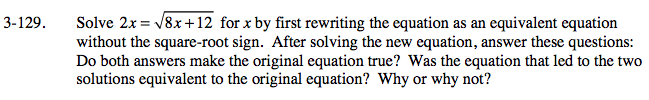### Home > A2C > Chapter 3 > Lesson 3.2.3 > Problem3-129

3-129.Square both sides of the equation.

4x2 = 8x + 12

Simplify and solve the resulting equation.

x2 − 2x − 3 = 0

x = 3. But, −1 does not work in the original equation.

Substitute each answer in the original equation. Only one of them will satisfy the equation.

$6=\sqrt{36}$

6 = 6

$-2=\sqrt{4}$

−2 = 2